## MP Board Class 9th Maths Solutions Chapter 13 Surface Areas and Volumes Ex 13.3

Assume π = $$\frac{22}{7}$$, unless stated otherwise.

Question 1.
Diameter of the base of a cone is 10.5 cm and its slant height is 10 cm. Find its curved surface area.
Solution:
Here, diameter of the base = 10.5 cm
Radius (r) = $$\frac{10.5}{2}$$
Slant height (l) = 10 cm
Curved surface area of the cone = πrl
= $$\frac{22}{7}$$ x $$\frac{10.5}{2}$$ x 10 cm2
= $$\frac{22}{7}$$ x $$\frac{10.5}{20}$$ x 10 cm2
= 11 x 15 x 1 cm2 = 165 cm2Question 2.
Find the total surface area of a cone, if its slant height is 21 m and diameter of its base is 24 m.
Solution:
Here, diameter = 24 m
Radius (r) = $$\frac{24}{2}$$ m = 12m
Slant height (l) = 21 m
Total surface area = πr (r + l)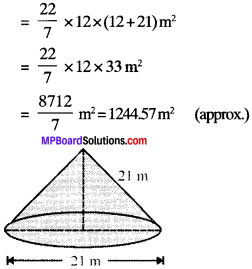Question 3.
Curved surface area of a cone is 308 cm2 and its slant height is 14 cm. Find:

1. radius of the base and
2. total surface area of the cone.

Solution:
Here, curved surface area = 308 cm2
Slant height (l) = 14 cm

1. Let the radius of the base be ‘r’ cm.
πrl = 308
$$\frac{22}{7}$$ x r x 14 = 308
r = $$\frac{308×7}{22×14}$$ = 7 cm
Thus, the required radius of the cone is 7 cm.

2. base area = πr2 = $$\frac{22}{7}$$ x 72 cm.
and curved surface area = 308 cm2 given
∴ Total surface area = [Curved surface area] + [Base area]
= 308 cm2 + 154 cm2 = 462 cm2Question 4.
A conical tent is 10 m high and the radius of its base is 24 m. Find

(i) slant height of the tent.
(ii) cost of the canvas required to make the tent, if the cost of 1 m2 canvas is ₹ 70.

Solution:
h = 10 m
r =24m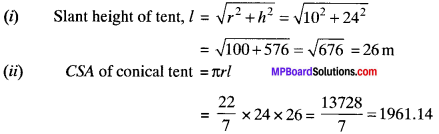Cost of canvas = CSA x Rate of canvas
= 1961.14 x 70
= ₹ 137279.8
= ₹ 137280.

Question 5.
What length of tarpaulin 3 m wide will be required to make conical tent of height 8 m and base radius 6 m? Assume that the extra length of material that will be required for stitching margins and wastage in cutting is approximately 20 cm. (Use π = 3.14)
Solution: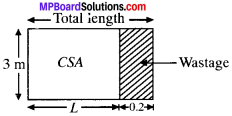h = 8m
r = 6m
Width of tarpaulin = 3 m
Extra length required = 20 cm = 0.2 m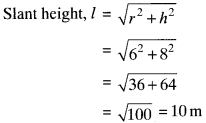CSA of conical tent = πrl
= 3.14 x 6 x 10 = 188.4 m2
CSA of conical tent = Area of tarpaulin
188.4 = l x 3 (Here l = length of tarpaulin)
l = $$\frac{188.4}{3}$$
Total length of tarpaulin required = 62.8 + 0.2 = 63 m

Question 6.
The slant height and base diameter of a conical tomb are 25 m and 14 m respectively. Find the cost of white-washing its curved surface at the rate of ₹ 210 per 100 m2.
Solution:
l = 25m
d = 14m
r = 7m
Rate of white washing = ₹ 210 per 100 m2
= $$\frac{210}{100}$$ = ₹ 2.10 per m2
CSA of conical tomb = πrl
= $$\frac{22}{7}$$ x 7 x 25 = 550 m2
Cost of white washing = Rate x CSA
= 2.10 x 550 (Rate per m2 = $$\frac{220}{100}$$)
= ₹ 1155

Question 7.
A joker’s cap is in the form of a right circular cone of base radius 7 cm and height 24 cm. Find the area of the sheet required to make 10 such caps.
Solution:
r = 7 cm
h = 24 cm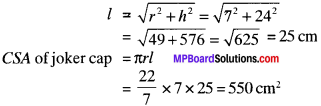Area of sheet required = CSA of 10 such caps = 550 x 10 = 5500 cm2.Question 8.
A bus stop is barricaded from the remaining part of the road, by using 50 hollow cones made of recycled cardboard. Each cone has a base diameter of 40 cm and height 1 m. If the outer side of each of the cones is to be painted and the cost of painting is ₹ 12 per m2 what will be cost of painting all these cones? (Use π = 3.14 and take $$\sqrt{1.04}$$ =1.02).
Solution:
d = 40 cm
r = 20 cm = 0.20 m
h = l m
Rate = ₹ 12 per m2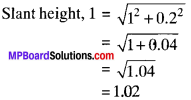= 1.02
CSA of cone = 3.14 x 0.2 x 1.02 = 0.64m2
Total area of 50 hollow cones= 50 x 0.64 = 32 m2
Cost of painting = 32 x 12 = ₹ 384
(Rate of painting = ₹ 12/m2)